# 一、二叉树的基本概念

## 1、二叉树的概念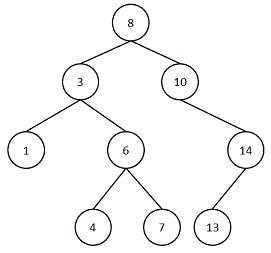## 2、一些常见的二叉树

• 满二叉树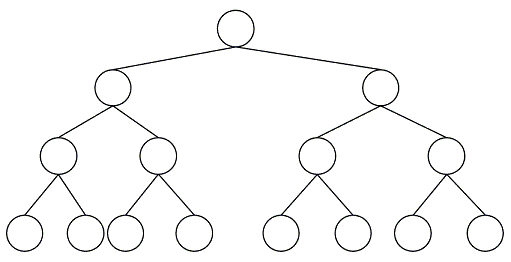• 完全二叉树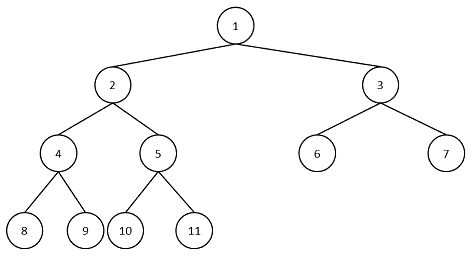## 3、二叉树的一些性质

• 在二叉树中，第 i $i$层上至多有2i1$2^{i-1}$个节点（ i1 $i\geq 1$
• 深度为 k $k$的二叉树至多有2k1$2^{k-1}$个节点（ k1 $k\geq 1$
• 对一棵二叉树，如果叶子节点的个数为 n0 $n_0$，度为 2 $2$的节点个数为n2$n_2$，则 n0=n2+1 $n_0 = n_2 + 1$
• 具有 n $n$个节点的完全二叉树的深度为log2n+1$\left \lfloor log _2 n \right \rfloor+1$

# 二、二叉树的基本操作

## 1、二叉树的存储结构• 顺序存储结构
• 链式存储结构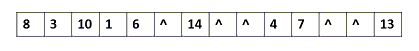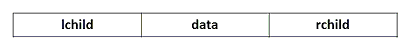typedef struct BiNode{
int data;// 数据域的值
struct BiNode *left;// 左孩子
struct BiNode *right;// 右孩子
}binode;

## 2、二叉树的遍历

• 前序遍历
• 中序遍历
• 后序遍历
• 层次遍历

// 先序遍历
void pre_order(binode *p){
if (p != NULL){
printf("%d\t", p->data);
pre_order(p->left);
pre_order(p->right);
}
}

// 中序遍历
void in_order(binode *p){
if (p != NULL){
in_order(p->left);
printf("%d\t", p->data);
in_order(p->right);
}
}

// 后序遍历
void post_order(binode *p){
if (p!= NULL){
post_order(p->left);
post_order(p->right);
printf("%d\t", p->data);
}
}

// 层次遍历
void lever_order(binode *p){
// 使用队列
list<binode *> t;
if (p != NULL){
t.push_back(p);
}

while (t.size() > 0){
printf("%d\t", (t.front())->data);
if ((t.front())->left != NULL){
t.push_back((t.front())->left);
}

if ((t.front())->right != NULL){
t.push_back((t.front())->right);
}
t.pop_front();
}
}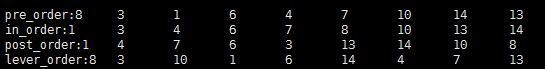# 参考文献

• 《大话数据结构》
• 《数据结构》(C语言版)
01-161万+05-231万+
09-301853
03-073607
01-022569
06-028618
07-231548
04-246197
02-282382
12-021354